# Prediction of Hourly Household Power ConsumptionPhoto by Markus Spiske on Unsplash

With the recent increase in smart meters across the residential sectors, we have large publicly available datasets. With such data, the power consumption of individual households can be tracked in almost real-time.

Such prediction can help power companies regulate their supply; also, the consumer can use this information to make better decisions both financially and environment-consciously.

In this project, we address this challenge by trying 4 different Machine learning Algorithms to do a comparative analysis to see which approach works best.

The 4 approaches used are:

• Auto Regression (AR Model)
• Support Vector Regression
• Linear Regression
• Random Forest

let’s move our discussion ahead towards the introduction of the project.

# Introduction

With the advent of new gadgets and a push towards greater electrification projects globally, power consumption is rising globally. (https://data.worldbank.org/indicator/EG.USE.ELEC.KH.PC).

Thus, we can also expect that household or residential power consumption is so on the rise. With greater access to global power consumption data, forecasting power consumption is an emerging challenge.

An accurate forecast can help both the consumer as well as the supplier side. For the consumer, a power forecast helps in financial planning as making more green choices overall. For the supplier, an accurate forecast will definitely help in supply regulation. Thus, such models can help to optimize the overall supply chain of the household power industry.

Since this is a fairly popular topic, multiple approaches from Neural Networks to Regression to Random Forest have been tried so far. Since this class’s scope is mostly limited to ML algorithms, the following discussion and overall project are based on ML algorithms only.

Though research has shown promise with DL Algorithms (Tae-Young Kim and Sung-Bae Cho, 2019), we shall not look into these algorithms for this project.

Four ML approaches show great promise with this type of forecasting. (Though, sometimes even a combination of multiple approaches is promising as well). These approaches are:

## Auto Regression

ARIMA models are, in theory, the most commonly used to forecast future values of time series data. Box and Jenkins first popularized the ARIMA model. It forecasts future values of a time series as a linear combination of its own past values and/or lags of the forecast errors (also called random shocks or innovations).

Box and Jenkins stated that these models do not involve independent variables but rather use the information in the series to generate forecasts. Therefore, ARIMA models depend on autocorrelation patterns in the series.

## Support Vector Regression (SVR)

SVR is an implementation of SVM to predict the continuous-valued output. In SVR, we use the margin the same as SVM. This margin around the target hyperplane signifies the amount of error that is tolerable in prediction.

This margin is defined by the parameter ϵ (in the above image)of the SVR. Instances that fall within the margin do not incur any cost; that’s why we refer to the loss as ‘epsilon-insensitive.’

SVR is an optimum margin regression algorithm that can work well even with non-linear data (with appropriate Kernel Tricks). (X. M. Zhang et al, 2018) have shown promising results on similar problems.

## Linear Regression

Linear Regression tries to fit a line in the data. If the target function is linear in nature, linear regression is fast with little correlation among the features.

The issue with Linear Regression is it’s sometimes too simple of a model to fit complicated real-world data properly.

Here in this work, this model has been used to have a baseline performance with a simple model before going on with more complicated ones.

## Random Forest

Random Forest Algorithm is based on a decision tree. A decision tree is very fast but is prone to overfitting.

Random Forest is used to addressing this specific reason only. Using a method called “Boot-Strap Aggregation” or Bagging, in short, we take random samples from the total dataset with replacement. Each random sample is then fitted to a decision tree. For output, we aggregate the result from each decision tree to get a single result.

As the number of trees in the forest increases, the more chance there is that trees have overlapping training sets. However, the advantage is that more votes are cast in the prediction process, decreasing the generalization error. It has been shown that as the number of trees increases, the accuracy approaches the theoretical limit of the forest (L. Breiman, 2001).

We now had the basic knowledge about the different models we are going to use here, so let’s move ahead with the preprocessing part of the dataset.

# Dataset Analysis and Visualization

The ‘Household Power Consumption’ dataset is a multivariate time series dataset that describes the electricity consumption for a single household over four years.

This dataset contains Minute by minute power consumption data for a Single household in Sceaux (7km of Paris, France) between December 2006 and November 2010 (47 months).

It is a multivariate series comprised of seven variables (besides the date and time), they are:

1. date: Date in format dd/mm/yyyy

2. time: time in format hh: mm: ss

3. global_active_power: household global minute-averaged active power (in kilowatt)

4. global_reactive_power: household global minute-averaged reactive power (in kilowatt)

5. voltage: minute-averaged voltage (in volt)

6. global_intensity: household global minute-averaged current intensity (in ampere)

7. sub_metering_1: energy sub-metering №1 (in watt-hour of active energy) corresponds to the kitchen, containing mainly a dishwasher, an oven, and a microwave (hot plates are not electric but gas-powered).

8. sub_metering_2: energy sub-metering №2 (in watt-hour of active energy) corresponds to the laundry room, containing a washing machine, a tumble-drier, a refrigerator, and a light.

9. sub_metering_3: energy sub-metering №3 (in watt-hour of active energy) corresponds to an electric water heater and an air-conditioner.

We can assume that Voltage*Current = Power should give a linear relationship between the two, confirmed below.

As Voltage and Current uniquely determine Active Power, these 2 are dropped as features, as there’s no use in using such a model.

Reactive Power is the total power loss due to all the appliances and is fairly randomly distributed.

But, the overall trend we can see is Reactive Power << Active Power. So, finally, active power is being considered only as this the power which is metered.

Now, the below plot shows Active Power vs. Sum of the 3 metered power. We see that active power is always > SUM (Metered Power). Thus, we conclude that metered power is a part of active power, and the rest is unmetered power.

For now, we considered Time as well as metered power to predict active power, as the sum of metered power gives a lower bound on active power. Further, you can extend it to make the model more robust and only include Time to predict.

Next, we investigate Time vs Active Power:

From the graph, we see that power consumption is highest at 9 pm, with the 2nd peak of around 9 am. On a monthly scale, we notice that August has, on avg, the lowest power consumption.

Annual Trends show that overall average power consumption is decreasing somewhat in this Time-Period.

Overall, we see that Time is a huge factor in determining power. Based on this, time and the 3-meter readings were chosen as features (except for Auto Regression Model), while Active Power has been chosen as the target Variable.

# Comparative Analysis and Proposed Plan

For the comparative analysis, the R2 parameter was used.

R2 is defined as:

Alternatively,

Thus, we can interpret the R2 score as the amount of variance in output explained by the model. E.g., an R2 score of 1 means the model explains 100% of the variance in the data. Hence we try to make the R2 score as high as possible. However, what the R2 score doesn’t tell us, is high good the individual model is.

So, we use the R2 score to compare between equivalent models and then check their RMSE ( shown below) to get an estimate of Regression Error.

Now let’s get to the model one by one.

## ARIMA Model

An ARIMA (p, d, q) model has three parameters. AR parameter ‘p’ represents the order of autoregressive process, I parameter ‘d’ represents the order of difference to obtain stationary series if the series are non-stationary, and MA parameter ‘q’ represents the order of moving average process. Autoregressive revolves around regressing the variable on its prior terms. The I parameter of the model is generally applied when the data in the sample are non-stationary. If the series are stationary, then d=0, and if the series is first-difference stationery, then d=1 and so forth. The moving-average parameter states that the variable linearly depends on the present and past values of a stochastic term.

Also, in the Autoregressive model, we relied on the Partial Autocorrelation Function, a.k.a PACF, and with Moving Averages, we rely on the Autocorrelation Function or the ACF for short. To get an idea about Lag's values we should take, we will plot the PACF and ACF plot.

here basically, we will focus on the AR model means we have to focus on to get and observe the AR parameter ‘p’ PACF (partial Autocorrelation plot); after getting the ‘p’ value and training our AR model on that prediction, we will get is:

it might look so unclear from the above plot so let’s have a zoom-in view into that.

This looks much better; as we can see, the blue color line shows the actual value, and the red line shows the predicted value. We can also observe that how close is the predicted to the actual value, although it still gives us an R2 score of 0.433 and RMSE of 32.77, which is not what we are looking for, so let’s try to see other models as well.

## Support Vector Regression and Linear Regression Model

In Support vector Regression, we use the margin same as SVM. This margin around the target hyperplane signifies the amount of error that is tolerable in prediction. The parameter ϵ of SVR defines this margin. Instances that fall within this margin do not incur any cost; that’s why we refer to the loss as ‘epsilon insensitive.’ And that’s why we are not concerned with the points that lie within this margin. The idea of SVR is to compute Linear Regression in a high dimension space where input data points are mapped using a nonlinear function.

In this approach, we have used 5 fold splitting for data to get a better insight into how the SVR and LR models behave for different folds.

So, let’s use SVR and Linear regression on the same folds of the data and compare their performance.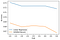R2 Score for different folds on SVM and LR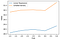RMSE for different folds on SVM and LR

As is evident from the plots, Linear Regression performs marginally better than SVR for forecasting the power consumption of a household. Now let’s move on to our last model, Random Forest, to see how well it holds its position compared to other models.

## Random Forest Model

Random Forest is a classifier that contains a number of decision trees on various subsets of the given dataset and takes the average to improve the predictive accuracy of that dataset.

A random forest combines output from multiple decision trees using bootstrapping or bagging. We randomly select multiple subsets of datapoints with replacements, train each model with 1 subset of data, and then take an average of the output of each model.

For our power consumption data, Random forest tends to consume a lot of disk space, and it’s important to restrict the maximum depth of the model. Otherwise, high depths can also lead to overfitting.

The number of trees also matters, with more trees taking more time but is more accurate. Here we have chosen max depth = 15, number of trees = 200, and then tried to plot the RMSE as

As we can see, the RMSE is pretty low for this model. Also, the R2 score we have got is R2=0.87, which is quite high and pretty good. Thus, overall we see that Random forest gives the best results at about R2=0.87, which is the best performance out of all models.

So this was the comparative analysis we have done with all models, and the table below shows us this comparison in a better way.

We can now conclude that Autoregression performed worst and Random Forest performed best with the maximum R2 score and minimum RMSE on our dataset. Now, what’s next?

After getting the best model in all four models, we can now focus solely on Random Forest for further analysis and prediction of power consumption.

## Prediction using Random Forest Model

Random Forest is made of multiple individual Regression Trees. Each Regression Tree was allowed to grow to a large number of nodes (and thus overfit). The output of each tree was taken into consideration by calculating their average. 50% of the total data was randomly assigned (with replacement) to every single tree for training purposes.

Hyperparameters: Number of Trees and Depth of each Tree.

The number of trees in a forest was fixed at 200 after observing performance, and we did a Grid Search on the Depth.

Parameters Used: 2 different types of model were implemented.

## 1st Model

Here, we used Time Data as well as Sub Metering Data into consideration. So, in that model, we predicted the total Power consumed (including wasted power) based on the available sub-metering readings as well as Time Data.

## 2nd Model

We recognize that calculating total power based on meter readings may not be efficient in real-world scenarios. So, this model was built to predict Hourly Power Consumption Based on time only.

As including sub-metering data would allow the model to train on more data and the total sub-meter readings provide a hard lower bound on the total power (when power wasted = 0), we expect that the 1st model should perform significantly better.

Let’s find it out.

## The model with Sub Metering Data

Plots for R2 scores: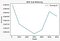For Training Dataset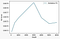For Validation Dataset

With Submeter data available, we see a validation R2 score of about 0.87, consistent with the value previously obtained during the comparison analysis. We also plot the error values to get an estimate of how good our model is. Those scores are presented below as :

Plots for RMSE: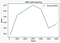For Training Dataset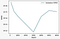For Validation Dataset

Overall, we see that the result is consistent in both train and validation sets with no sign of over/underfitting. Also, RMSE is about 19–20 KW throughout.

## The Model with Time Data only

Plots for R2 scores: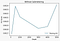For Training Dataset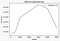For Validation Dataset

Here, we see that for the validation set, the R2 score is significantly lower at around 0.48 and, We also see that the model actually starts overfitting after a maximum depth of 4000.

Plots for RMSE: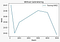For Training Dataset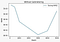For Validation Dataset

With average Global Power in the data about 65.5 KW, this RMSE is about 60% of the mean power in case of only time data and about 30% of the mean power when sub-metering is also included. So, now it’s time to jump to a conclusion.

# Conclusion

This project looked at ML Based implementation of Short Term Power Forecasting. Where we tried Random Forest, SVM, Linear Regression as well as Auto Regression approaches. Of these, Random Forest showed the most promise. So, that algorithm was looked into in greater detail.

Overall, we saw that this model is great in predicting Total Wasted Power (when metered power is known) with an R2 score of about 0.87, but not so great with predicting absolute power (R2 score of about 0.48) consumption-based on just an hour of the day.

The RMSE values are still high, so other approaches should be tried out as well. Since this data is temporal in nature, RNN based approaches like LSTM, etc., can be looked into as well.

Overall, this project article will allow you to gain an insight into the world of Real Data Forecasting and its various challenges like incomplete data, inconsistent data, etc.

# Contributors

## Team Work

As we can see, we have implemented 4 models in this project and did an in-depth analysis based on these models. We, as a group of 3 members, have divided these models among us and analyzed them on an individual basis. Every one of us gave his best and equal effort for the completion of the Project.

Team Members are: Arnav Yadav, Souradip Sanyal and Vaibhav Bhat

Arnav Yadav (https://www.linkedin.com/in/iamarnavyadav/) ARIMA model analysis and prepared the presentation as well as this blog.

Souradip Sanyal (https://www.linkedin.com/in/souradip-sanyal-0889b73a/) Random Forest Analysis and prepared the report.

Vaibhav Bhat (https://www.linkedin.com/in/vaibhav-bhat-55aaa415b/) SVR as well as Linear regression analysis and wrote the code for the final model.

The task of the project report and presentation was a team effort.

We are especially thanking our course project instructor (Dr. Tanmoy) to give this wonderful opportunity to us, below is his social media profiles link

Twitter: @Tanmoy_chak

## References:

L. Breiman, 2001. Random forests, Machine Learning 45.1: 5–32

Tae-Young Kim and Sung-Bae Cho, 2019. Predicting residential energy consumption using CNN-LSTM neural networks. Energy, Volume 182: 72–81. https://doi.org/10.1016/j.energy.2019.05.230

X. M. Zhang, K. Grolinger, M. A. M. Capretz and L. Seewald, 2018. Forecasting Residential Energy Consumption: Single Household Perspective. 17th IEEE International Conference on Machine Learning and Applications (ICMLA), Orlando, FL, 2018: 110–117, doi: 10.1109/ICMLA.2018.00024

## Analytics Vidhya

Analytics Vidhya is a community of Analytics and Data…

## Sign up for Analytics Vidhya News Bytes

### By Analytics Vidhya

Latest news from Analytics Vidhya on our Hackathons and some of our best articles! Take a look.

By signing up, you will create a Medium account if you don’t already have one. Review our Privacy Policy for more information about our privacy practices.

Check your inbox
Medium sent you an email at to complete your subscription.

## Analytics Vidhya

Analytics Vidhya is a community of Analytics and Data Science professionals. We are building the next-gen data science ecosystem https://www.analyticsvidhya.com

Written by

## Arnav Yadav

M-Tech Student at IIIT Delhi## Analytics Vidhya

Analytics Vidhya is a community of Analytics and Data Science professionals. We are building the next-gen data science ecosystem https://www.analyticsvidhya.com

## More From Medium

Medium is an open platform where 170 million readers come to find insightful and dynamic thinking. Here, expert and undiscovered voices alike dive into the heart of any topic and bring new ideas to the surface. Learn more

Follow the writers, publications, and topics that matter to you, and you’ll see them on your homepage and in your inbox. Explore

If you have a story to tell, knowledge to share, or a perspective to offer — welcome home. It’s easy and free to post your thinking on any topic. Write on Medium